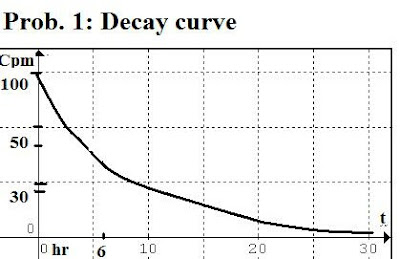## Monday, August 25, 2014

### Solutions to Basic Atomic Physics (Part 3)1)     The sketch of the decay curve for Problem (1) is shown above, and we note it conforms to the half life (T½) = 6h, already computed.

2) The decay curve is shown for Problem 2 (accompanying sketch graph two above) and we see on inspection that since the counts per minute decreases from 360 to 180 in ONE half life (e.g. 1(T½)). The critical aspect to note is that the time is given in units of HALF LIVES not hours! Thus, since 1(T½)= 30 mins. = 1800s then (T½)= 1800 s. This is confirmed from the curve since the 180 counts/min decreases to 90 in 2 half-lives, and this decreases to 45 in 3 half lives and so on.

The activity A = ln 2/(T½) = 0.693/ 1800s

= 3.85 x 10-4 s

3)A = 5.6 x 10-7 /s.

(T½) = ln 2/ A = 0.693/ (5.6 x 10-7 /s)

(T½) = 1.24 x 106 s = 14.3 days

4) Solution:

Given:    dN/dt = - lN

So that: l   =    1/N | dN/dt |   =  10 -15 (6.00 x 1011/s)

l   =  6.00 x 10-4 s-1

A = - lN   =   - ( 6.00 x 10-4 s-1 )( 10 15 )=   -  6.00 x 1011/s  i.e.

6.00 x 1011    decays per second

c)  Half life: T½  =  ln 2/l    = 0.693/ l =

0.693/ (6.00 x  10-4 s-1)

=  1160 s  (or 19.3 minutes)

5) Solution (a): we note that for a multi-electron atom we have for the Bohr –atom energy:

En =  - 13.6 (Z eff)2/ n2    where:   Z eff  = (Z – 10)

Note that Z eff   is the atomic number (Z =11)  minus the number of electrons between the nucleus and the electron being considered. Since 10 electrons separate via ionization the last  remaining sodium electron (e.g. the electron that occupies  the 3s sub-level of the n= 3 principal level  (electron arrangement for sodium is: (1s2, 2s2, 2p6, 3s1)).

Then (given the 1st line corresponds to n = 3):

En (eV) =  - 13.6 (Z- 10)2 / (3) 2

En  = -13.6 eV (1)/9 = 13.6 eV/9 = 1.51 eV

Now, the energy is related to the wavelength (l )  in nm by:

El  = 1.99 x 10 -16  J nm

To get the energy in Joules, we use the fact that:

1 eV = 1.6 x 10 -19J  so 1.51 eV = 1.51 eV(1.6 x 10 -19J /eV)

E = 2.41 x 10 -19  J   Then:

l  = 1.99 x 10 -16  J nm  / 2.41 x 10 -19 J    = 825 nm

(b)   The voltage of 10 4 V leads to an energy:

E = qV = (1. 6 x 10 -19C) (10 4 V) =  1. 6 x 10 -15 J

6)   SolutionThe activity of a radio-nuclide is given as:

A =  Ao  exp (-lt)

Where  Ao is the decay rate at time t = 0, and A refers to the decay rate at some time t thereafter.

a) The decay constant  l = 0.693/ T1/2   =

0.693/ 883612800s

Or: l =  7.84 x 10 -10

b) The activity A  after 1 hour (3600 s) is given by:

A =  Ao  exp (-lt)  = 1. 1 x 10 10  decays/sec (exp (-lt) )

Where (lt)  =  (7.84 x 10 - 10 ) (3600 s) = 2.82 x 10 - 6

Then:

Ao  exp (-lt)  = 1. 1 x 10 10  /s [exp (-2.82 x 10 - 6)] =

1. 1 x 10 10  /s [1.00000] =  1. 1 x 10 10  decays/sec

After two hours (t = 7200s ):

(lt)  =  (7.84 x 10 - 10 ) (7200 s) = 5.64 x 10 - 6

Ao  exp (-lt)  = 1. 1 x 10 10  /s [exp (-5.64 x 10 - 6)] =

1. 1 x 10 10  /s [1.0000] =  1. 1 x 10 10  decays/sec

After 49 years, T = 1. 54 x 10 9  sec

lt  =  (7.84 x 10 - 10 ) (1. 54 x 10 9   s) = 1.20

Ao  exp (-lt)  = 1. 1 x 10 10  /s [exp (- 1.20)]

=1. 1 x 10 10  /s (0.301) =     3.31 x 10 9   decays/sec